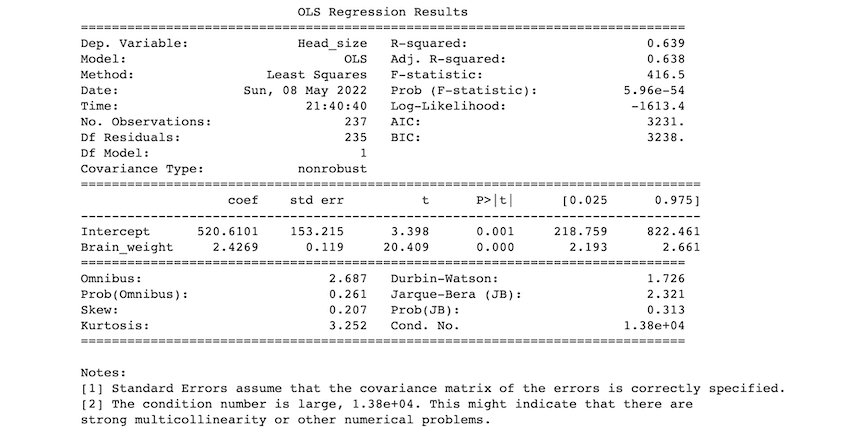Open In App

# How to Get Regression Model Summary from Scikit-Learn

In this article, we are going to see how to get a regression model summary from sci-kit learn.

It can be done in these ways:

• Scikit-learn Packages
• Stats model package

### Example 1: Using scikit-learn.

You may want to extract a summary of a regression model created in Python with Scikit-learn. Scikit-learn does not have many built-in functions for analyzing the summary of a regression model because it is generally used for prediction. Scikit learn has different attributes and methods to get the model summary.

We imported the necessary packages. Then the iris dataset is loaded from sklearn.datasets. And feature and target arrays are created then test and train sets are created using the train_test_split() method and the simple linear regression model is created then train data is fitted into the model, and predictions are carried out on the test set using .predict() method.

## Python3

 `# Import packages``from` `sklearn.linear_model ``import` `LinearRegression``from` `sklearn.model_selection ``import` `train_test_split``from` `sklearn.datasets ``import` `load_iris`` ` `# Load the data``irisData ``=` `load_iris()`` ` `# Create feature and target arrays``X ``=` `irisData.data``y ``=` `irisData.target`` ` `# Split data into train and test sets``X_train, X_test, y_train, y_test ``=` `train_test_split(``    ``X, y, test_size``=``0.2``, random_state``=``42``)`` ` `model ``=` `LinearRegression()`` ` `model.fit(X_train, y_train)`` ` `# predicting on the X_test data set``print``(model.predict(X_test))`` ` `# summary of the model``print``(``'model intercept :'``, model.intercept_)``print``(``'model coefficients : '``, model.coef_)``print``(``'Model score : '``, model.score(X, y))`

Output:

[ 1.23071715 -0.04010441  2.21970287  1.34966889  1.28429336  0.02248402

1.05726124  1.82403704  1.36824643  1.06766437  1.70031437 -0.07357413

-0.15562919 -0.06569402 -0.02128628  1.39659966  2.00022876  1.04812731

1.28102792  1.97283506  0.03184612  1.59830192  0.09450931  1.91807547

1.83296682  1.87877315  1.78781234  2.03362373  0.03594506  0.02619043]

model intercept : 0.2525275898181484

model coefficients :  [-0.11633479 -0.05977785  0.25491375  0.54759598]

Model score :  0.9299538012397455

### Example 2: Using the summary() method of Stats model package

In this method, we use the statsmodels. formula.api package. If you want to extract a summary of a regression model in Python, you should use the statsmodels package. The code below demonstrates how to use this package to fit the same multiple linear regression model as in the earlier example and obtain the model summary.

## Python3

 `# import packages``import` `numpy as np``import` `pandas as pd``import` `statsmodels.formula.api as smf`` ` `# loading the csv file``df ``=` `pd.read_csv(``'headbrain1.csv'``)``print``(df.head())`` ` `# fitting the model``df.columns ``=` `[``'Head_size'``, ``'Brain_weight'``]``model ``=` `smf.ols(formula``=``'Head_size ~ Brain_weight'``,``                ``data``=``df).fit()`` ` `# model summary``print``(model.summary())`

Output:Description of some of the terms in the table :

• R-squared value: The R-squared value ranges from 0 to 1. An R-squared of 100% indicates that changes in the independent variable completely explain all changes in the dependent variable (s). If the r-squared value is 1, it indicates a perfect fit. The r-squared value in our example is 0.638.
• F-statistic: The F-statistic compares the combined effect of all variables. Simply put, if your alpha level is greater than your p-value, you should reject the null hypothesis.
• coef: the coefficients of the regression equation’s independent variables.

Our predictions:

If we use 0.05 as our significance level, we reject the null hypothesis and accept the alternative hypothesis as p< 0.05. As a result, we can conclude that there is a relation between head size and brain weight.